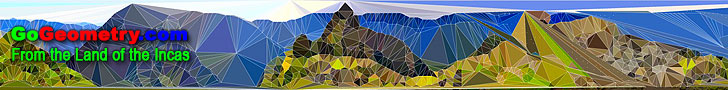# Recent additions (Page 18), Online LearningGo Geometry from the Land of the Incas - Recent Additions (Page 18) Problem 585. Cyclic Quadrilateral, Diagonals, Circumcenters, Circumcircles, Concurrency. Problem 584. Cyclic Quadrilateral, Diagonals, Circumcenters, Circumcircles, Parallelograms. Problem 583. Quadrilateral, Diagonals, Circumcenters, Parallelogram, Octagon, Areas. Problem 582. Quadrilateral, Diagonals, Incenters, Metric Relations. Problem 581. Volume of two Triangular Pyramids, Equal Trihedral Angle, Product of three Edges. Problem 580. Triangles, Equal Angle, Transversal, Product of Sides, Areas. Problem 579. Quadrilateral, Diagonals, Triangles, Areas. Problem 578. Circle, Perpendicular Chords, Midpoints, Curvilinear Triangle, Area, 90 Degrees. Problem 577. Isosceles triangle, Midpoint, Perpendicular, Concyclic points, Circle. Problem 576. Centroid, Triangle, Quadrilateral, Parallel, Measurement. Go to Page: Previous | 1 | 2 | 3 | 4 | 5 | 6 | 7 | 8 | 9 | 10 | 11 | 12 | 13 | 14 | 15 | 16 | 17 | 18 | 19 | 20 | 21 | 22 | 23 | 24 | 25 | 26 | 27 | 28 | 29 | 30 | 31 | 32 | 33 | 34 | 35 | 36 | 37 | 38 | 39 | 40 | 41 | 42 | 43 | 44 | 45 | 46 | 47 | 48 | 49 | 50 | Next

 Home | GoGeometry Education | Geometry | Search | Email